• import numpy as np np.deg2rad(45) Out: 0.7853981633974483python
• 1.#由numpy转换为torch数据 numpy [[0 1 2] [3 4 5]] torch_data=torch.from_numpy(np_data) 2.#由tensor数据转换为numpy数据 torch tensor([[0, 1, 2], [3, 4, 5]], dtype=torch.int32) tensor2array=torch_...
np_data=np.arange(6).reshape((2,3))

1.#由numpy转换为torch数据
numpy [[0 1 2]
[3 4 5]]

torch_data=torch.from_numpy(np_data)

2.#由tensor数据转换为numpy数据
torch tensor([[0, 1, 2],
[3, 4, 5]], dtype=torch.int32)

tensor2array=torch_data.numpy()

tensor2array [[0 1 2]
[3 4 5]]

3.abs函数
data=[-1,-2,1,2]
tensor=torch.FloatTensor(data)
print(
'\nabs',
'\nnumpy',np.abs(data),
'\ntorch',torch.abs(tensor)
)

abs
numpy [1 2 1 2]
torch tensor([1., 2., 1., 2.])

torch.abs要处理的数据必须是tensor，而不能是list。torch .abs等同于numpy.abs函数
4.#sin如abs一样
data=[-1,-2,1,2]
tensor=torch.FloatTensor(data)
print(
'\nsin',
'\nnumpy',np.sin(data),
'\ntorch',torch.sin(tensor)
)

sin
numpy [-0.84147098 -0.90929743  0.84147098  0.90929743]
torch tensor([-0.8415, -0.9093,  0.8415,  0.9093])

其他的函数也如同numpy类似，需要可以百度查询 5.#矩阵相乘
data=[[1,2],[3,4]]
tensor=torch.FloatTensor(data)
print(
'\nnumpy矩阵相乘:',np.matmul(data,data),
'\ntorch矩阵相乘:',torch.mm(tensor,tensor)
)

numpy矩阵相乘: [[ 7 10]
[15 22]]
torch矩阵相乘: tensor([[ 7., 10.],
[15., 22.]])

展开全文python 人工智能 pytorch 深度学习
• 本节主要介绍numpy中的运算函数，有些需要记住并熟练的使用，主要分为三类：数学运算、算数运算、统计运算。 1. 数学函数 1.1 三角函数 因为这些函数都比较简单，不需要用实例说明。下面列出了函数的名称以及功能...
本节主要介绍numpy中的运算函数，有些需要记住并熟练的使用，主要分为三类：数学运算、算数运算、统计运算。
1. 数学函数
1.1 三角函数
因为这些函数都比较简单，不需要用实例说明。下面列出了函数的名称以及功能描述，使用时直接查表就可以。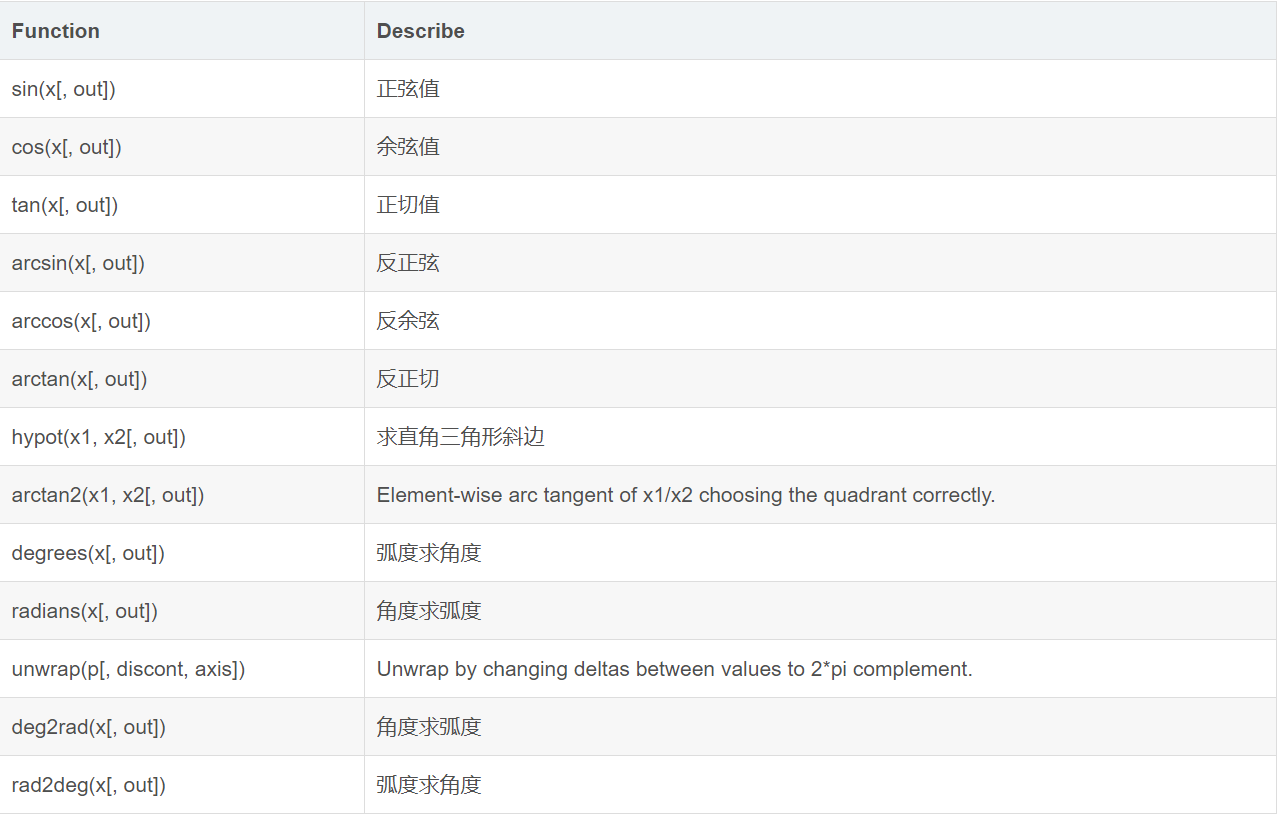1.2 双曲线函数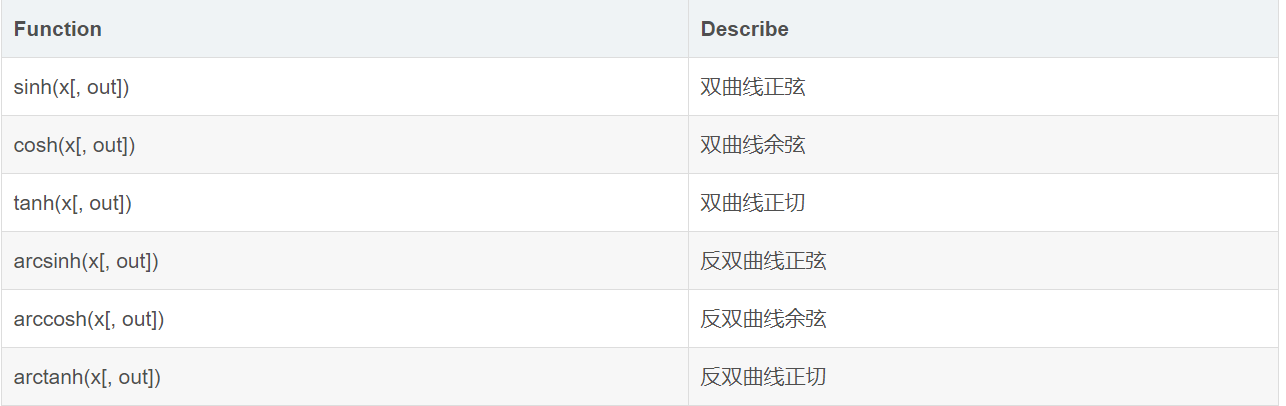1.3 舍入函数
舍入函数主要涉及到一些精度变换的函数，例如保留小数位数、向下取整、向上取整等。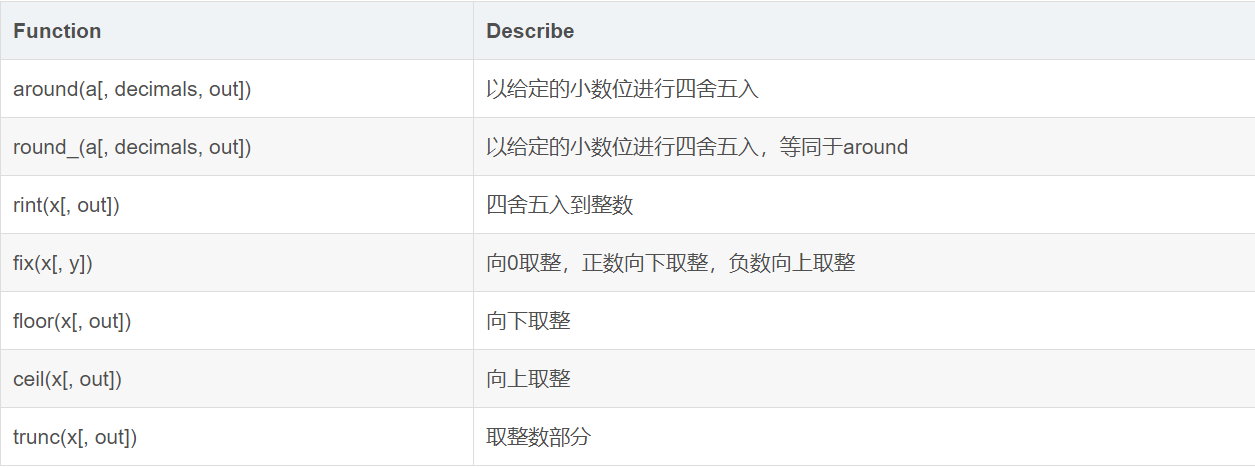2. 运算函数
2.1 指数、对数函数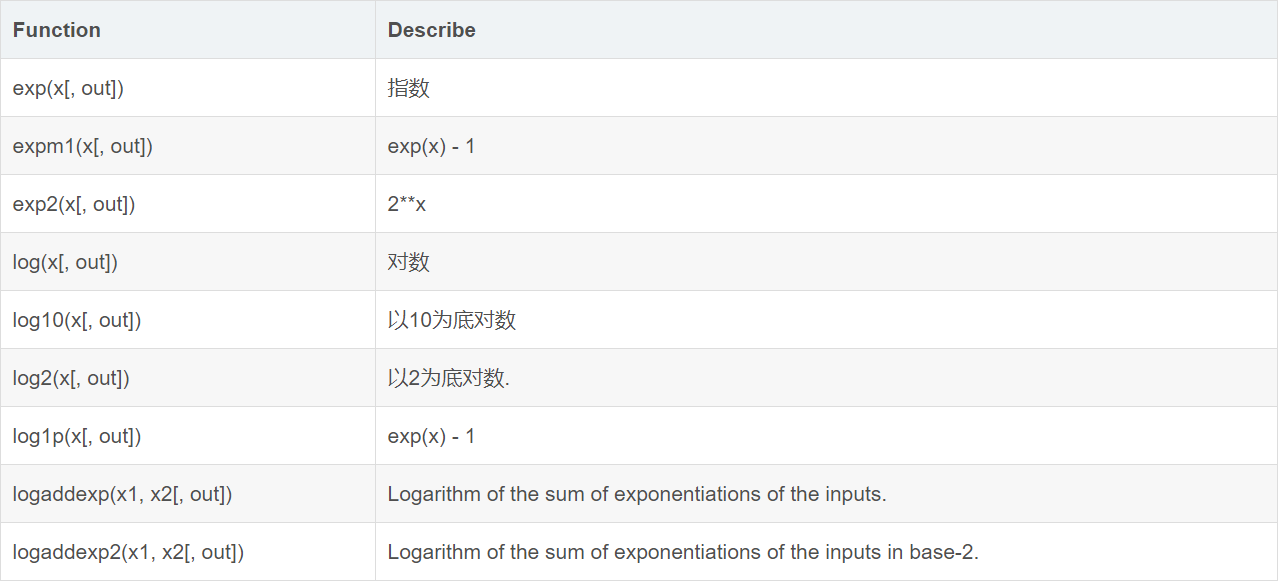2.2 四则运算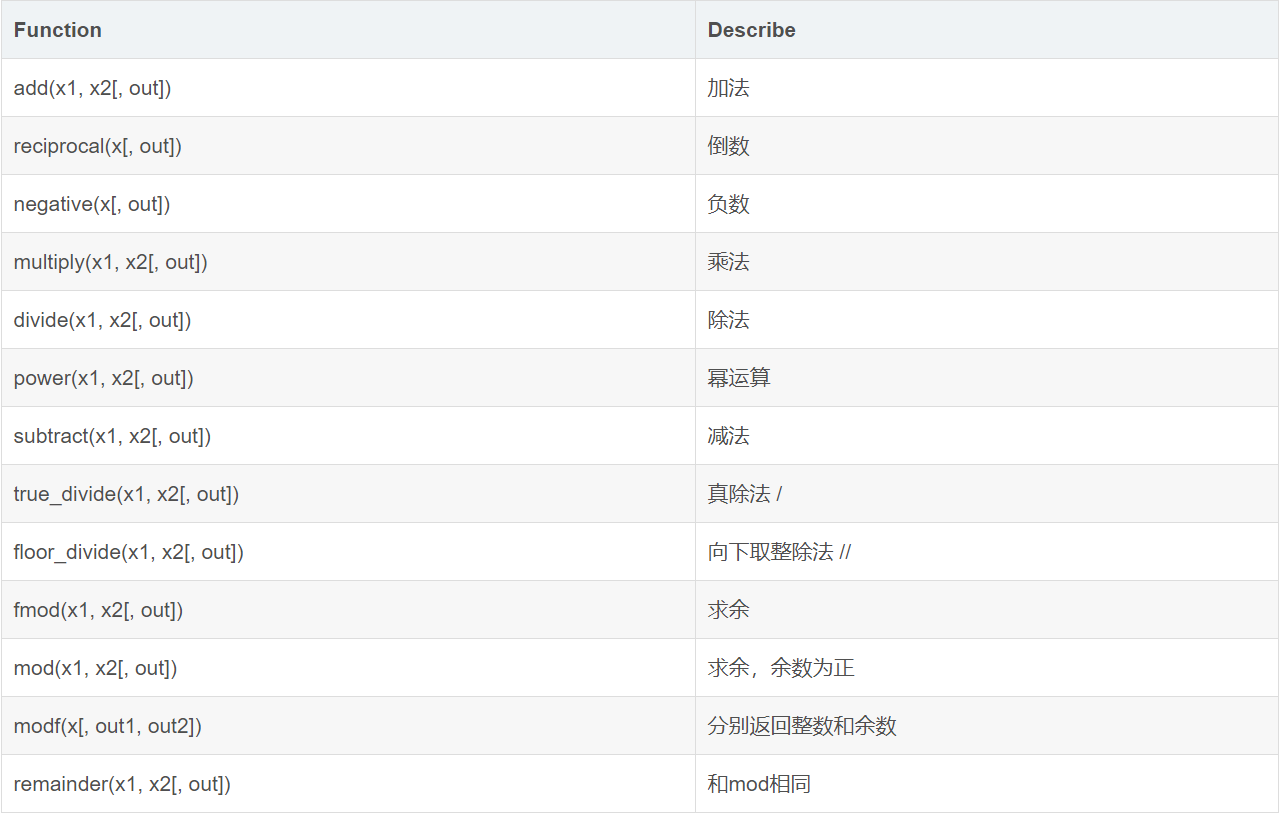2.3 高级运算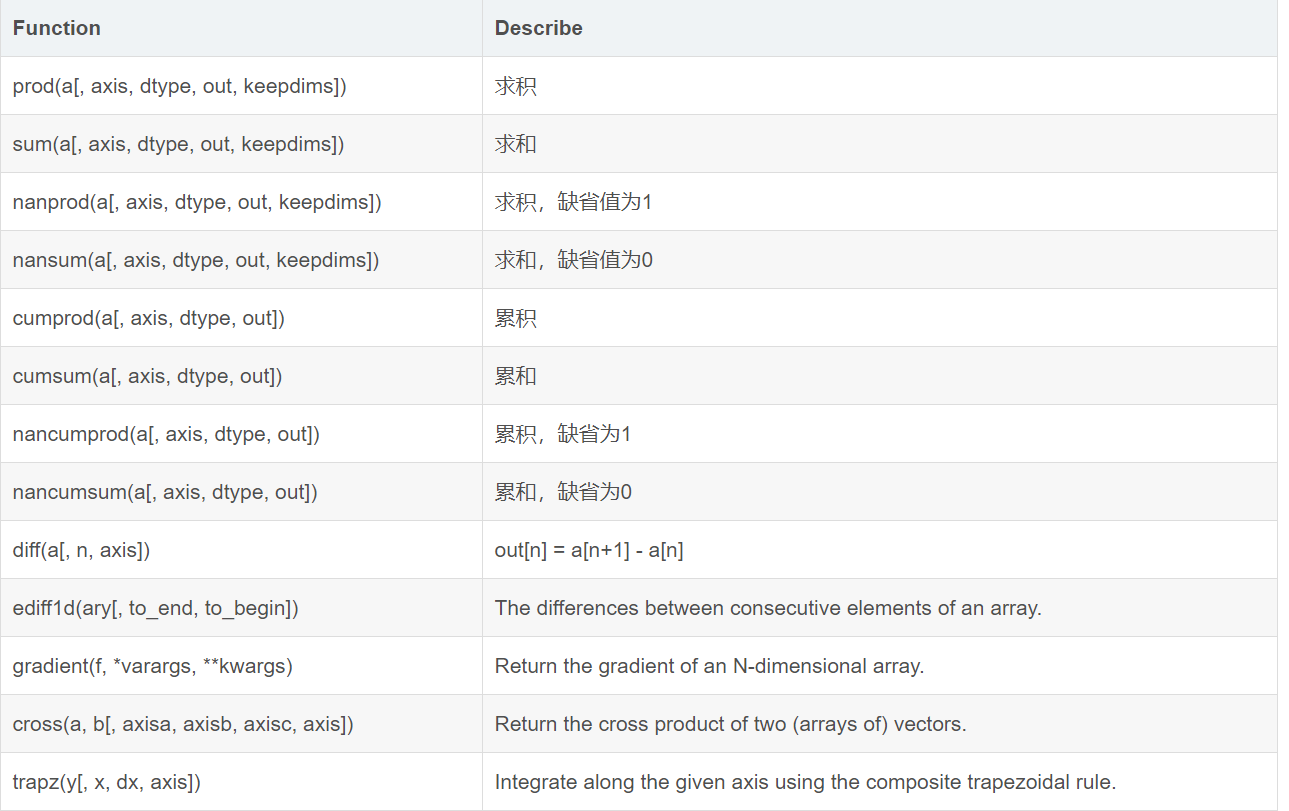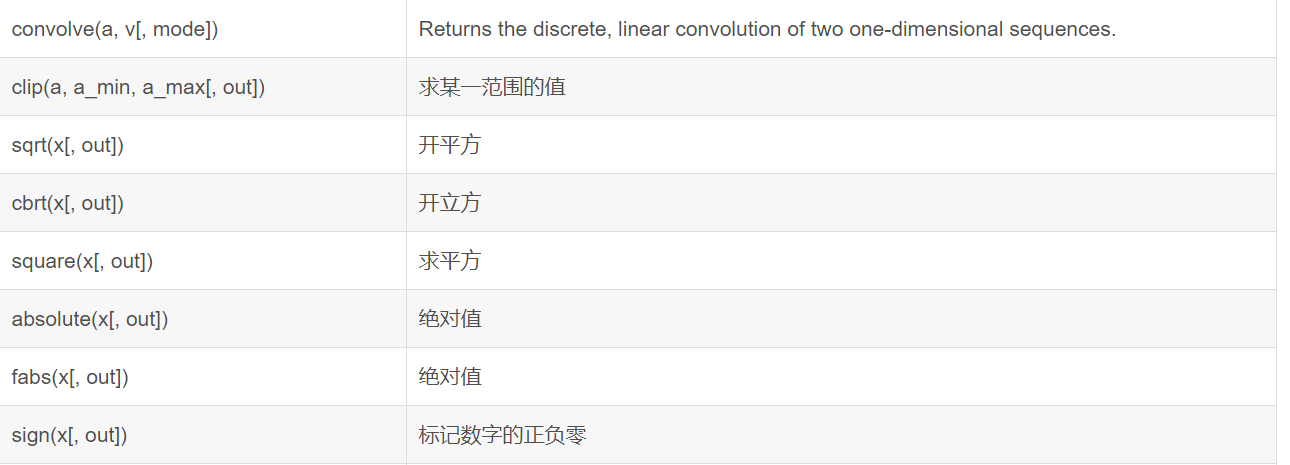3. 统计运算
3.1 排序统计函数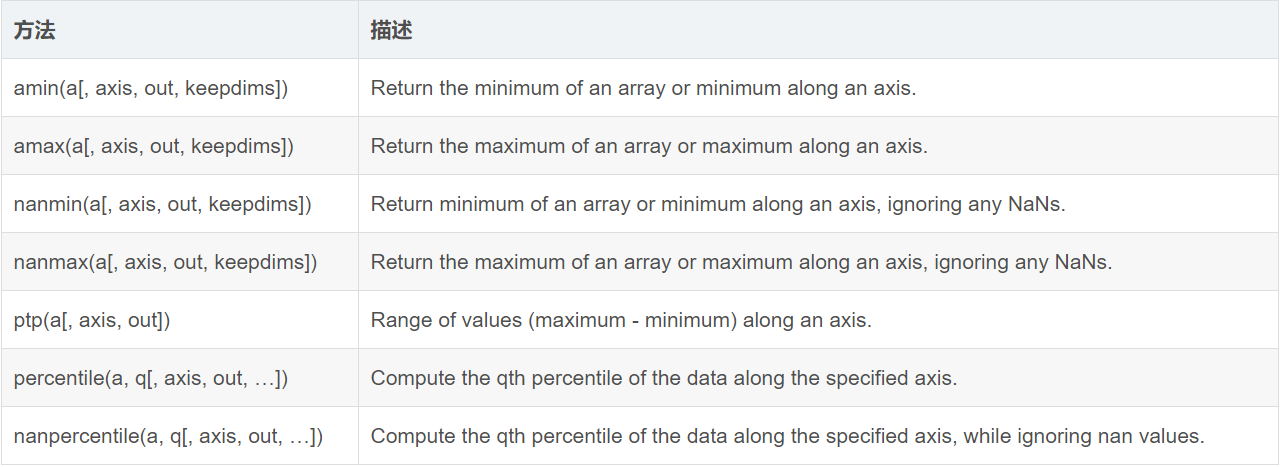3.2 均数和方差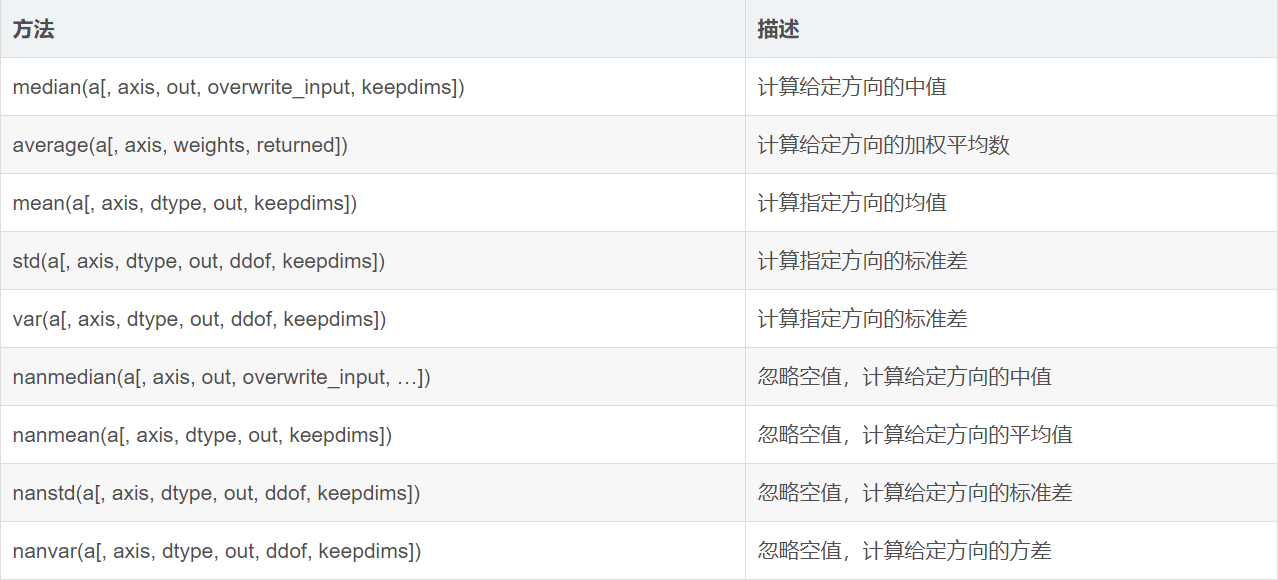3.3 相关性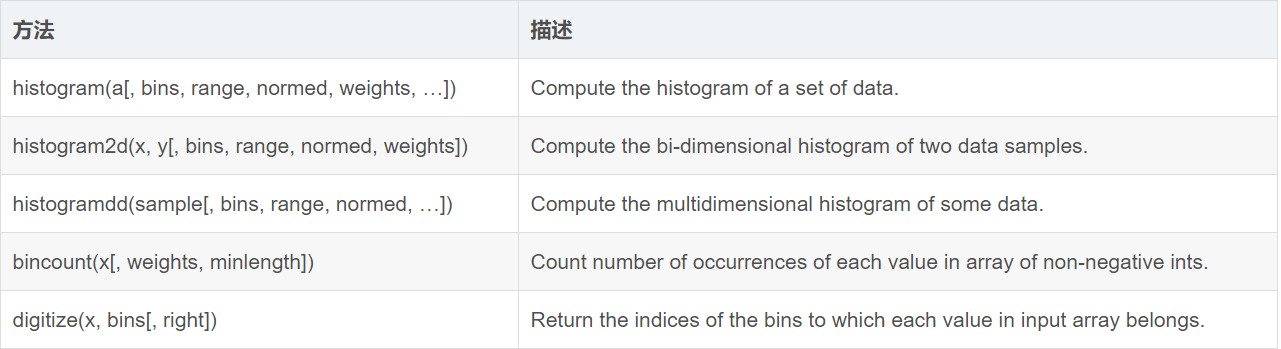这些函数都是直接使用即可，基本没有需要注意和解释的地方，使用的时候查表就行。
展开全文numpy指数函数 numpy统计函数
•python...